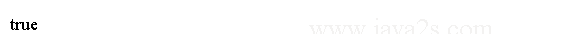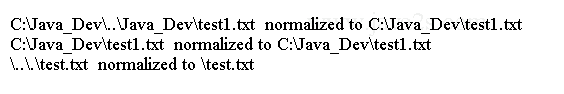# Java IO Tutorial - Java Paths Operations

## Comparing Paths

We can compare two Path objects for equality based on their textual representation.

The equals() method tests for the equality of two Path objects by comparing their string forms.

Whether the equality test is case-sensitive depends on the file system.

The following code shows how to compare Windows paths:

```import java.nio.file.Path;
import java.nio.file.Paths;
//w  w w.  j a v  a2s  .  c  om
public class Main {
public static void main(String[] args) throws Exception {
Path  p1  = Paths.get("C:\\Java_Dev\\test1.txt");
Path  p2  = Paths.get("C:\\Java_Dev\\LUCI1.TXT");
Path  p3  = Paths.get("C:\\Java_Dev\\..\\Java_Dev\\test1.txt");
boolean b1  = p1.equals(p2);
System.out.println(b1);
boolean b2  = p1.equals(p3);
System.out.println(b2);
}
}
```

The equals() method compares two paths textually without resolving the actual file references and it does not test a Path for existence in the file system.

The Path interface implements the java.lang.Comparable interface. We can use its compareTo() method to compare it with another Path object textually.

The compareTo() method returns an int value.

• 0 - when the two paths are equal
• less than 0 - the path is less than the specified path
• greater than 0 - the path is greater than the specified path

The code above generates the following result.## Example

The following code shows how to use the compareTo() method:

```import java.nio.file.Path;
import java.nio.file.Paths;
/*w w  w .  j a  v a  2  s.  c o  m*/
public class Main {
public static void main(String[] args) throws Exception {
Path  p1  = Paths.get("C:\\Java_Dev\\test1.txt");
Path  p2  = Paths.get("C:\\Java_Dev\\Test1.txt");
Path  p3  = Paths.get("C:\\Java_Dev\\..\\Java_Dev\\test1.txt");
int v1  = p1.compareTo(p2);
System.out.println(v1);
int v2  = p1.compareTo(p3);
System.out.println(v2);
}
}
```

We can use the endsWith() and startsWith() methods to test if a path ends with and starts with a given path, respectively.

The code above generates the following result.## Example 2

The following code shows how to use endsWith() and startsWith() methods with paths.

```import java.nio.file.Path;
import java.nio.file.Paths;
/*  w  w w  . j a  va2 s .  c  o  m*/
public class Main {
public static void main(String[] args) throws Exception {
Path p1 = Paths.get("C:\\Java_Dev\\test1.txt");
Path p2 = Paths.get("test1.txt");
Path p3 = Paths.get("Java_Dev\\test1.txt");
Path p4 = Paths.get(".txt");

// Using endsWith()
boolean b1 = p1.endsWith(p2);
System.out.println(b1);
boolean b2 = p1.endsWith(p3);
boolean b3 = p1.endsWith(p4);

// Using startsWith()
Path p5 = Paths.get("C:\\");
Path p6 = Paths.get("C:\\Java_Dev");
Path p7 = Paths.get("C:\\Java_Dev");

boolean b4 = p1.startsWith(p5);
boolean b5 = p1.startsWith(p6);
boolean b6 = p1.startsWith(p7);
}
}
```

The code above generates the following result.## Example 3

The following code demonstrates how the isSameFile() method works. It checks If Two Paths Will Locate the Same File.

```import java.io.IOException;
import java.nio.file.Files;
import java.nio.file.Path;
import java.nio.file.Paths;
/*w  w w . j a va 2 s .co m*/
public class Main {
public static void main(String[] args) {
// Assume that C:\Java_Dev\test1.txt file exists
Path p1 = Paths.get("C:\\Java_Dev\\test1.txt");
Path p2 = Paths.get("C:\\Java_Dev\\..\\Java_Dev\\test1.txt");

// Assume that C:\abc.txt file does not exist
Path p3 = Paths.get("C:\\abc.txt");
Path p4 = Paths.get("C:\\abc.txt");

try {
boolean isSame = Files.isSameFile(p1, p2);
System.out.println("p1 and  p2  are   the   same:  " + isSame);

isSame = Files.isSameFile(p3, p4);
System.out.println("p3 and  p4  are   the   same:  " + isSame);
}catch (IOException e) {
e.printStackTrace();
}
}
}
```

## Normalizing Paths

The normalize() method from the Path interface returns a Path after removing dot dot characters.

This method does not access the file system.

The following code shows some examples of normalizing paths on Windows.

```import java.nio.file.Path;
import java.nio.file.Paths;
// w ww .j a v a  2  s .  c  o m
public class Main {
public static void main(String[] args) throws Exception {
Path p1 = Paths.get("C:\\Java_Dev\\..\\\\Java_Dev\\test1.txt");
Path p1n = p1.normalize();
System.out.println(p1 + "  normalized to " + p1n);

Path p2 = Paths.get("C:\\Java_Dev\\test1.txt");
Path p2n = p2.normalize();
System.out.println(p2 + "  normalized to " + p2n);

Path p3 = Paths.get("\\..\\.\\test.txt");
Path p3n = p3.normalize();
System.out.println(p3 + "  normalized to " + p3n);
}
}
```

The code above generates the following result.## Resolving Paths

We can combine two paths using the resolve(Path p) method of the Path interface.

If the specified path is an absolute path, it returns the specified path. It returns the path if the specified path is an empty path.

In other cases, it simply combines the two paths and returns the result, so the returned path ends with the specified path.

The following code has some examples of resolving paths.

```import java.nio.file.Path;
import java.nio.file.Paths;
//from   ww  w  . j  a  v a2s  .  c  o  m
public class Main {
public static void main(String[] args) throws Exception {
Path p1 = Paths.get("C:\\Java_Dev");
Path p2 = Paths.get("test1.txt");
System.out.println(p1.resolve(p2));

Path p3 = Paths.get("C:\\test.txt");
System.out.println(p1.resolve(p3));

Path p4 = Paths.get("");
System.out.println(p1.resolve(p4));

Path p5 = Paths.get("Java_Dev\\Test");
Path p6 = Paths.get("test4.txt");
System.out.println(p5.resolve(p6));
}
}
```

The code above generates the following result.## Relativizing Paths

The relativize(Path p) method of the Path interface gets a relative path for a given path against another path.

The following code has some examples of getting relative paths.

```import java.nio.file.Path;
import java.nio.file.Paths;
/*  w  ww . j av  a2 s  .  co  m*/
public class Main {
public static void main(String[] args) throws Exception {
Path p1 = Paths.get("Java_Dev");
Path p2 = Paths.get("Java_Dev", "recent", "Test");
System.out.println(p1.relativize(p2));
System.out.println(p2.relativize(p1));

Path p3 = Paths.get("Abc");
Path p4 = Paths.get("Def");
System.out.println(p3.relativize(p4));
System.out.println(p4.relativize(p3));
}
}
```

The code above generates the following result.